# Fisher-F-distribution

(Redirected from Snedecor distribution)-distribution, Fisher–Snedecor distribution, Snedecor distribution

A continuous probability distribution concentrated on $(0,\infty)$ with density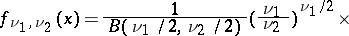(1)where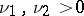are parameters, andis the beta-function. Forit is a unimodal positive asymmetric distribution with mode at the point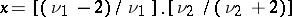. Its mathematical expectation and variance are, respectively, equal toand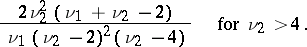The Fisher-distribution reduces to a beta-distribution of the second kind (a type-VI distribution in Pearson's classification). It can be regarded as the distribution of a random variable represented in the form of the quotientwhere the independent random variables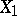and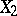have gamma-distributions (cf. Gamma-distribution) with parameters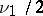and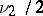, respectively. The distribution function for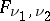can be expressed in terms of the distribution function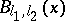of the beta-distribution: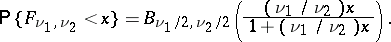(2)

This relation is used for calculating the values of the Fisher-distribution by means of tables of the beta-distribution. If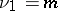andare integers, then the Fisher-distribution withanddegrees of freedom is the distribution of the-quotient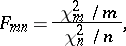(3)

whereand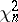are independent random variables with "chi-squared" distributions (cf. Chi-squared distribution) withanddegrees of freedom, respectively.

The Fisher-distribution plays a fundamental role in mathematical statistics and appears in the first place as the distribution of the quotient of two sample variances. Namely, let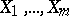and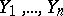be samples from normal populations with parametersand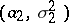. The expressions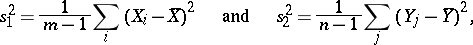where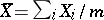,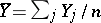, serve as estimators of the variances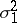and. Then the so-called dispersion proportionhas a Fisher-distribution withand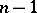degrees of freedom under the hypothesis that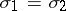(in this capacity the Fisher-distribution is also called the distribution of the dispersion proportion). The-test is based on the statistic, and it is used, in particular, for testing the hypothesis that the variances of two populations are equal, in the analysis of variance, regression analysis and multi-dimensional statistical analysis.

The universality of the Fisher-distribution is underlined by its connections with other distributions. For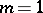the square of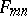in (3) has a Student distribution withdegrees of freedom. There are a number of approximations of the Fisher-distribution using the normal and "chi-squared" distributions.

The introduction of the Fisher-distribution in the analysis of variance is connected with the name of R.A. Fisher (1924), although Fisher himself used a quantityfor the dispersion proportion, connected withby the relation. The distribution ofwas tabulated by Fisher, and the Fisher-distribution by G. Snedecor (1937). At present the simpler Fisher-distribution is preferred, making use of its connection with the beta-distribution and tables of the incomplete beta-function.